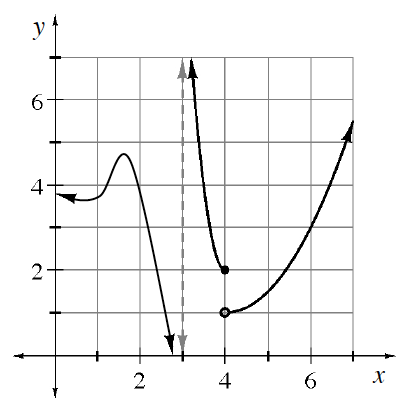### Home > APCALC > Chapter 2 > Lesson 2.4.1 > Problem2-143

2-143.

​Review the three conditions of continuity. Then, examine the graph below and determine at which values of $x$ the function is not continuous. Explain which condition the function fails at each discontinuity.

The three conditions of continuity are:

$1. \lim \limits_{x \rightarrow a} f(x) \text{ exists} \\ 2. f(a) \text{ exists}\\ 3. \lim \limits_{x \rightarrow a} f(x) =f(a)$